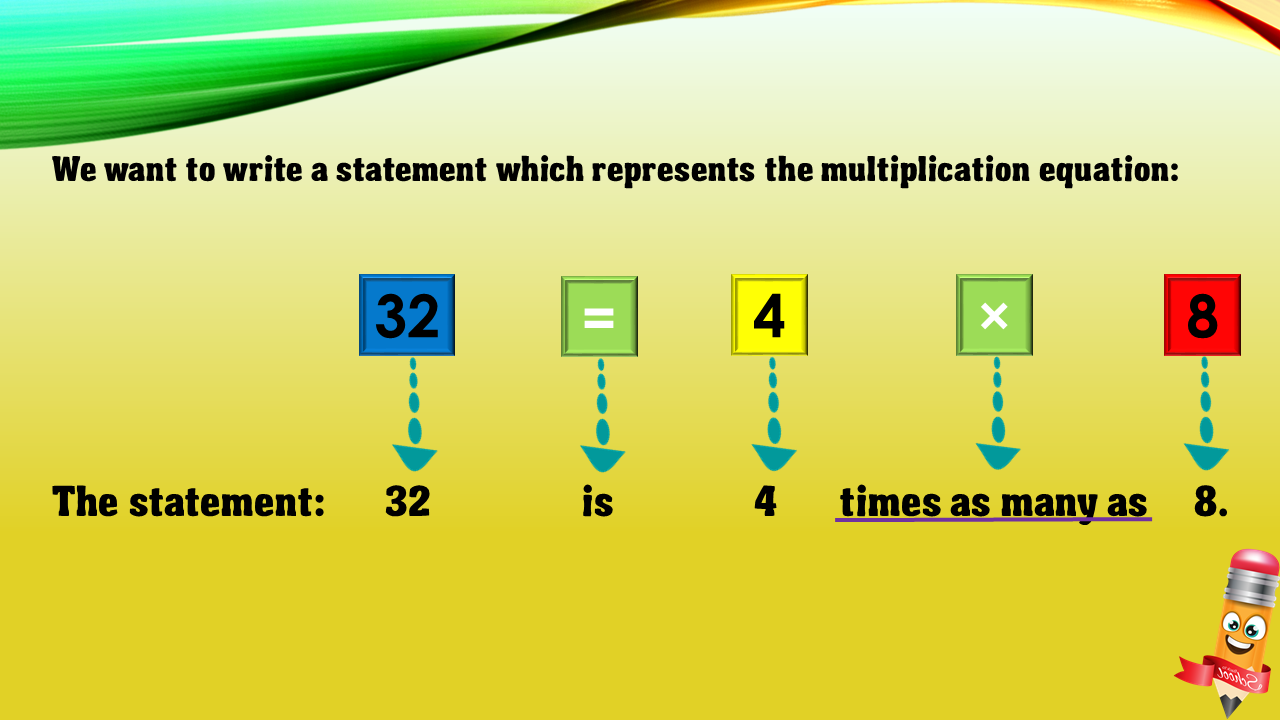1
visibility

Choose one statement which represents the equation: 32 = 4×8 ?

• A

32 times as many as 4 is 8.

• B

32 is 4 times as many as 8.

• C

32 is 4 more than 8.

• D

4 is 8 less than 32.